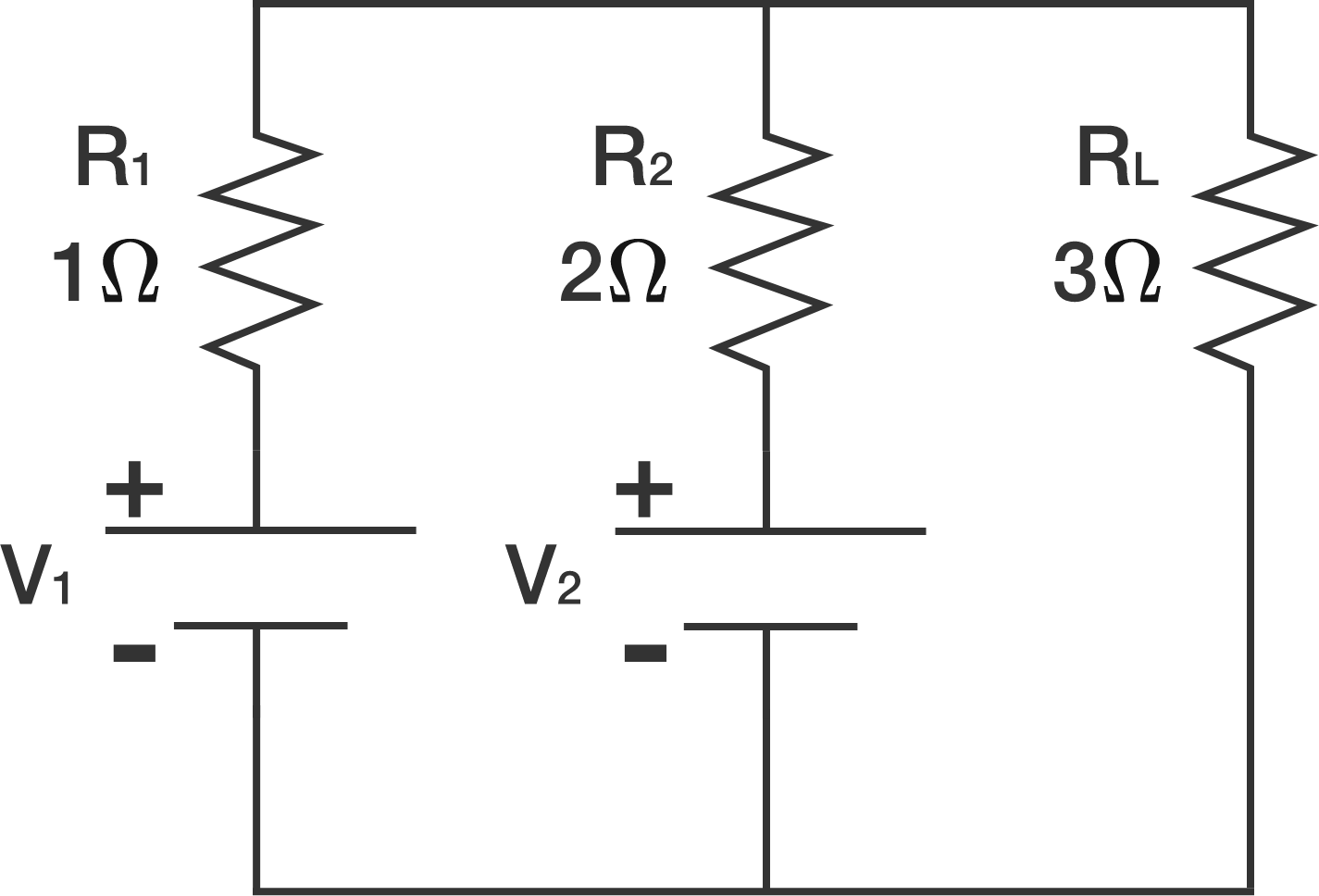# Optimizing a CircuitThe DC circuit above consists of two voltage sources $V_1$ and $V_2$ with internal resistances $R_1 = 1 \Omega$ and $R_2 = 2 \Omega$ respectively. There is a load $R_L = 3 \Omega$ connected in parallel with the sources.

$V_1$ and $V_2$ are variable quantities.

Let $P_1$, $P_2$, and $P_L$ be the amounts of power in watts dissipated by $R_1$, $R_2$, and $R_L$ respectively.

Given that $P_L = 10$ watts, determine the minimum possible value of $P_1 + P_2 + P_L$ to 1 decimal place.

×

Problem Loading...

Note Loading...

Set Loading...